# Operational Amplifiers

### What are Operational Amplifiers?

Operational amplifiers are the basic building blocks of Analogue electronic circuits. They are linear devices with all properties of a DC amplifier. We can use external resistors or capacitors to the Op Amp is many different ways to make them different forms of amplifies such as Inverting amplifier, Non inverting amplifier, Voltage follower, Comparator, Differential amplifier, Summing amplifier, Integrator etc. OPAMPs may be single, dual, quad etc. OPAMPs like CA3130, CA3140, TL0 71, LM311 etc have excellent performance with very low input current and voltage. The ideal Op Amp has three important terminals in addition to other terminals. The input terminals are Inverting input and Non inverting input. The third terminal is the output which can sink and source current and voltage. The output signal is the amplifiers gain multiplied by the value of the input signal.

### 5 Ideal characters of an Op Amp:

1. Open Loop gain

Open loop gain is the gain of the Op Amp without a positive or negative feedback. An ideal OP Amp should have an infinite open loop gain but typically it range between 20,000 and 2, 00000.

2. Input impedance

It is the ratio of the input voltage to input current. It should be infinite without any leakage of current from the supply to the inputs. But there will be a few Pico ampere current leakages in most Op Amps.

3. Output impedance

The ideal Op Amp should have zero output impedance without any internal resistance. So that it can supply full current to the load connected to the output.

4. Band width

The ideal Op Amp should have an infinite frequency response so that it can amplify any frequency from DC signals to the highest AC frequencies. But most Op Amps have limited bandwidth.

5. Offset

The output of the Op Amp should be zero when the voltage difference between the inputs is zero. But in most Op Amps, the output will not be zero when off but there will be a minute voltage from it.

### OPAMP Pin Configuration: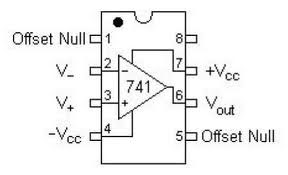In a typical Op Amp there will be 8 pins. These are

Pin1 – Offset Null

Pin2 – Inverting input INV

Pin3 – Non inverting input Non-INV

Pin4 – Ground- Negative supply

Pin5 – Offset Null

Pin6 – Output

Pin7 – Positive supply

Pin8 – Strobe

### 4 types of gain in OPAMPs:

Voltage gain – Voltage in and voltage out

Current gain – Current in and Current out

Transconductance – Voltage in and Current out

Trans resistance – Current in and voltage out

### Working of an Operational Amplifier:

Here we used an operational amplifier of LM358. Usually a non-inverting input has to be given to a biasing and the inverting input is the real amplifier; connected this to a feedback of 60k resister from output to the input. And a resister 10k is connected in series with a capacitor and a supply of 1V sine wave is given to the circuit, now we will see how gain will be governed by R2/R1=60k/10k=6 gain, then the output is 6V. If we change the gain by 40 then the output is 4V of sine wave.

### Video on Working of Operational Amplifier

Normally, it is a dual power supply amplifier, it easily configured to a single power supply by the use of a resister network. In this, resister R3 and R4 place a voltage of half of the supply voltage across the non-inverting input which causes the output voltage to also be half of the supply voltage forming a sort of bias voltage resisters R3 and R4 can be any value from 1k to 100k but in all cases they should be equal. An additional, 1 F capacitor has been added to the non-inverting input to reduce noise caused by the configuration. The use of coupling capacitors for input and output is required for this configuration.

### 3 OPAMP applications:

1. Amplification

The amplified output signal from the Op Amp is the difference between the two input signals.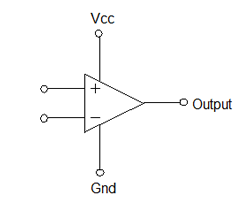The diagram shown above is the Op Amp simple connection. If both the inputs are supplied with the same voltage, the Op Amp will then takes the difference between the two voltages and it will be 0. The Op Amp will multiply this with its gain 1,000,000 so the output voltage is 0. When 2 volts is given to one input and 1 volt in the other, then the Op Amp will takes its difference and multiply with the gain. That is 1 volt x 1,000,000. But this gain is very high so to reduce the gain, feedback from the output to the input is usually done through a resistor.

### Inverting Amplifier: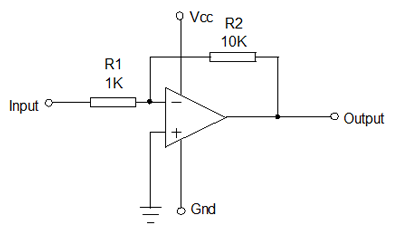The circuit shown above is an inverting amplifier with the Non inverting input connected to the ground. Two resistors R1 and R2 are connected in the circuit in such a fashion that R1 feeds the input signal while R2 returns the output to the Inverting input. Here when the input signal is positive the output will be negative and vice versa. The voltage change at the output relative to the input depends on the ratio of the resistors R1 and R2. R1 is selected as 1K and R2 as 10K. If the input receives 1 volt, then there will be 1 mA current through R1 and the output will have to become – 10 volts in order to supply 1 mA current through R2 and to maintain zero voltage at the Inverting input. Therefore the voltage gain is R2/R1. That is 10K/1K = 10

### Non-inverting Amplifier: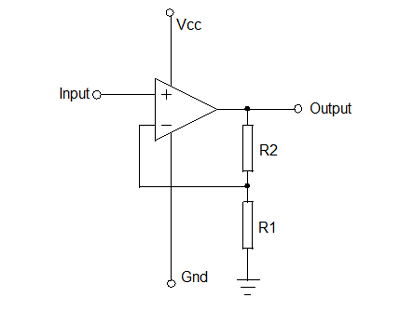The circuit shown above is a Non inverting amplifier. Here the Non inverting input receives the signal while the Inverting input is connected between R2 and R1. When the input signal moves either positive or negative, the output will be in phase and keeps the voltage at the inverting input same as that of Non inverting input. The voltage gain in this case will be always higher than 1 so (1+R2/R1).

2. Voltage Follower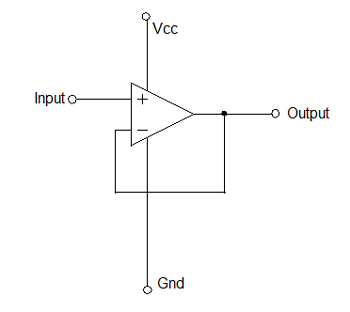The circuit above is a voltage follower. Here it provides high input impedance, low output impedance .When the input voltage changes, the output and the inverting input will change equally.

3. Comparator

Operational amplifier compares the voltage applied at one input to the voltage applied at the other input. Any difference between the voltages ever if it is small drives the op-amp into saturation. When the voltages supplied to both the inputs are of the same magnitude and the same polarity, then the op-amp output is 0Volts.

A comparator produces limited output voltages which can easily interface with digital logic, even though compatibility needs to be verified.

### Video on Operational Amplifier as a Comparator Circuit Diagram

Here we have an op-amp used as a comparator with the inverting and non-inverting terminals and connected some potential divider and meter to them and a voltmeter at the output and LED to the output. The basic formula for comparator is that when’+’ is more than the ‘–‘then the output is high (one), otherwise output is zero. When the voltage on the negative input is the below the reference voltage, the output is high and when the negative input goes above the voltage on the positive, the output goes to low.

### 3 Requirements for OPAMPs:

1. Offset Nulling

Most of the OPAMP has an Offset voltage at the output even if the input voltages are same. To make the output to zero voltage, the offset nulling method is used. In most Op-Amps there is a small offset because of their inherent property and results from the mismatches in the input bias arrangement. So a small output voltage is available at the output of some Op-amps even if the input signal is zero. This drawback can be rectified by providing a small offset voltage to the inputs. This is known as the Input Offset voltage. To remove or Null the Offset, most Op-Amps have two pins to enable the offset nulling. For this, a Pot or Preset with a typical value of 100K should be connected between the pins 1 and 5 with its Wiper to the ground. By adjusting the preset, output can be set at Zero voltage.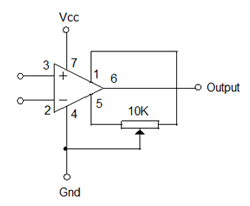2. Strobing or Phase compensation

Op-Amps may become unstable sometimes and to make them stable for the entire frequency bands a Cap is usually connected between its Strobe pin 8 and pin1. Usually a 47pF disc capacitor is added for phase compensation so that the OpAmp will remain stable. This is most important if the OpAmp is used as a sensitive Amplifier.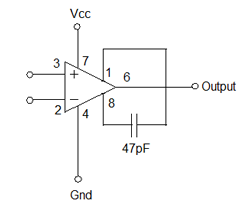3. Feedback

As you know, the Op-Amp has very high level of amplification typically around 1,000,00 times. Suppose the Op-Amp has 10,000 gain, then the Op-Amp will amplify the difference of voltage in its Non inverting input ( V+) and Inverting input ( V-). So the output voltage V out is
10,000 x (V+ – V- )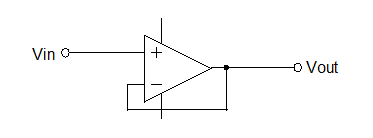In the diagram, the signal is applied to the Non inverting input and in Inverting input is connected to the output. So V+ = V in and V- = Vout. Therefore Vout = 10,000 x (Vin – Vout). Hence the output voltage is almost equal to the input voltage.

Now let us see how the Feedback works. Simply adding a resistor between the inverting input and the output will reduce the gain considerably. By taking a fraction of the output voltage to the inverting input can reduce the amplification considerably.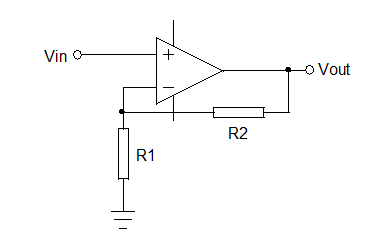As per the earlier equation, V out = 10,000 x ( V+ – V-). But here a feedback resistor is added. So Here V+ is Vin and V- is R1.R1+R2 x V out.  Therefore V out is 10,000 x ( Vin – R1.R1+R2xVout).  So V out = R1+R2.R1x Vin

### Negative Feedback:

Here the output of the Op-Amp is connected to its Inverting ( – ) input, thus the output is fed back to the input so as to reach an equilibrium. Thus the input signal at the Non Inverting (+) input will be reflected at the output. The Op-amp with the negative feedback will drive its output to level necessary and hence the voltage difference between its inverting and non inverting inputs will be almost zero.

### Positive Feedback:

Here the output voltage is fed back to the Non inverting (+) input. The input signal is fed to the Inverting input. In positive feedback design, if the Inverting input is connected to ground, then the output voltage from the Op-amp will depends on the magnitude and polarity of voltage at the Non inverting input. When the input voltage is positive, then the output of the Op-Amp will be positive and this positive voltage will be fed to the Non inverting input resulting in a full positive output. If the input voltage is negative, then the condition will be reversed.

### An Application of Operational Amplifiers – Audio Preamplifier

Filters and pre-amplifiers:

Power amplifiers will come after the pre-amplifiers and before the speakers. Modern CD and DVD players don’t need pre-amplifiers. They need volume control and source selectors. By using switching controls and passive volume we can avoid pre-amplifiers.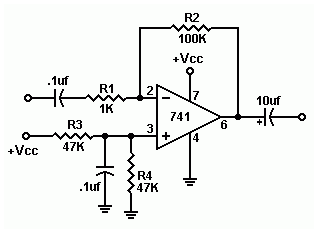Let us have a brief about Audio power amplifiers

The power amplifier is a component that can drive the loud speakers by converting the low-level signal into large signal. The job of power amplifiers is producing relatively high voltage and high current. Commonly the range of voltage gain is in between 20 to 30. The power amplifiers are having very low output resistance.

Specifications of Audio Power Amplifier

• Maximum output power:

The output voltage is independent of load, for both small and large signals. The given voltage applied to load causes twice the amount of current. Hence twice the amount of power will be delivered. The power rating is the continuous average sine wave power such that the power can be measured by employing a sine wave whose RMS voltage is measured on long term basis.

• Frequency response:

The frequency response must extend full audio band 20 Hz to 20 KHz. The tolerance to the frequency response is ±3db. The conventional way of specifying the bandwidth is an amplifier is down by 3db from the nominal 0db.

• Noise:

The power amplifiers should produce low noise when the power amplifiers are using with high frequencies. The noise parameter may be weighted or un-weighted. Un-weighted noise will be specified over 20 KHz-bandwidth. Based on the ear’s sensitivity weighted noise specification will be taken into consideration. The weighted noise measurement tends to attenuate the noise at higher frequencies hence weighted noise measurement is quite better than un-weighted noise measurement.

• Distortion:

Total harmonic distortion is the common distortion usually specified at different frequencies. This will be specified at a power level which is given with the power amplifier driving load impedance.